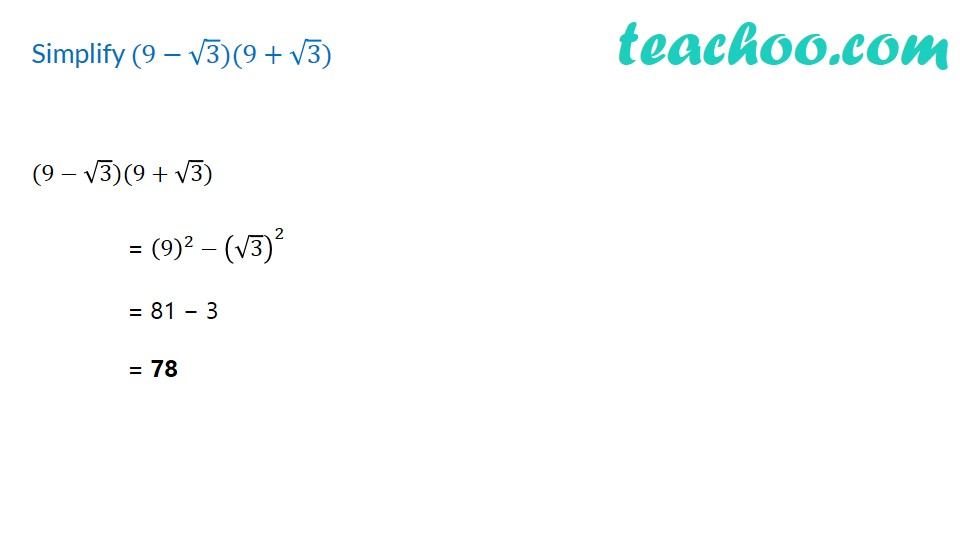1. Chapter 1 Class 9 Number Systems (Term 1)
2. Concept wise
3. Rationalising

Transcript

Simplify (9−√3)(9+√3) (9−√3)(9+√3) = (9)^2−(√3)^2 = 81 − 3 = 78

Rationalising